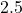## Suppose Gabor, a scuba diver, is at a depth of 15m15m. Assume that: The air pressure in his air tract is the same as the net water pressure

Question

Suppose Gabor, a scuba diver, is at a depth of 15m15m. Assume that: The air pressure in his air tract is the same as the net water pressure at this depth. This prevents water from coming in through his nose. The temperature of the air is constant (body temperature). The air acts as an ideal gas. Salt water has an average density of around 1.03 g/cm3g/cm3, which translates to an increase in pressure of 1.00 atmatm for every 10.0 mm of depth below the surface. Therefore, for example, at 10.0 mm, the net pressure is 2.00 atmatm. What is the ratio of the molar concentration of gases in Gabor’s lungs at the depth of 15 meters to that at the surface

in progress 0
5 months 2021-08-15T13:35:40+00:00 1 Answers 12 views 0

## Answers ( )

The ration of molar concentration is “2.5“.

Explanation:

The given values are:

Average density of salt water,

=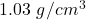Net pressure,

=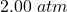Increase in pressure,

=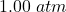Now,

The under water pressure will be:

=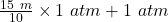=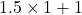=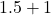=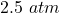hence,

The ratio will be:

=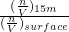or,

=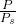=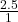=